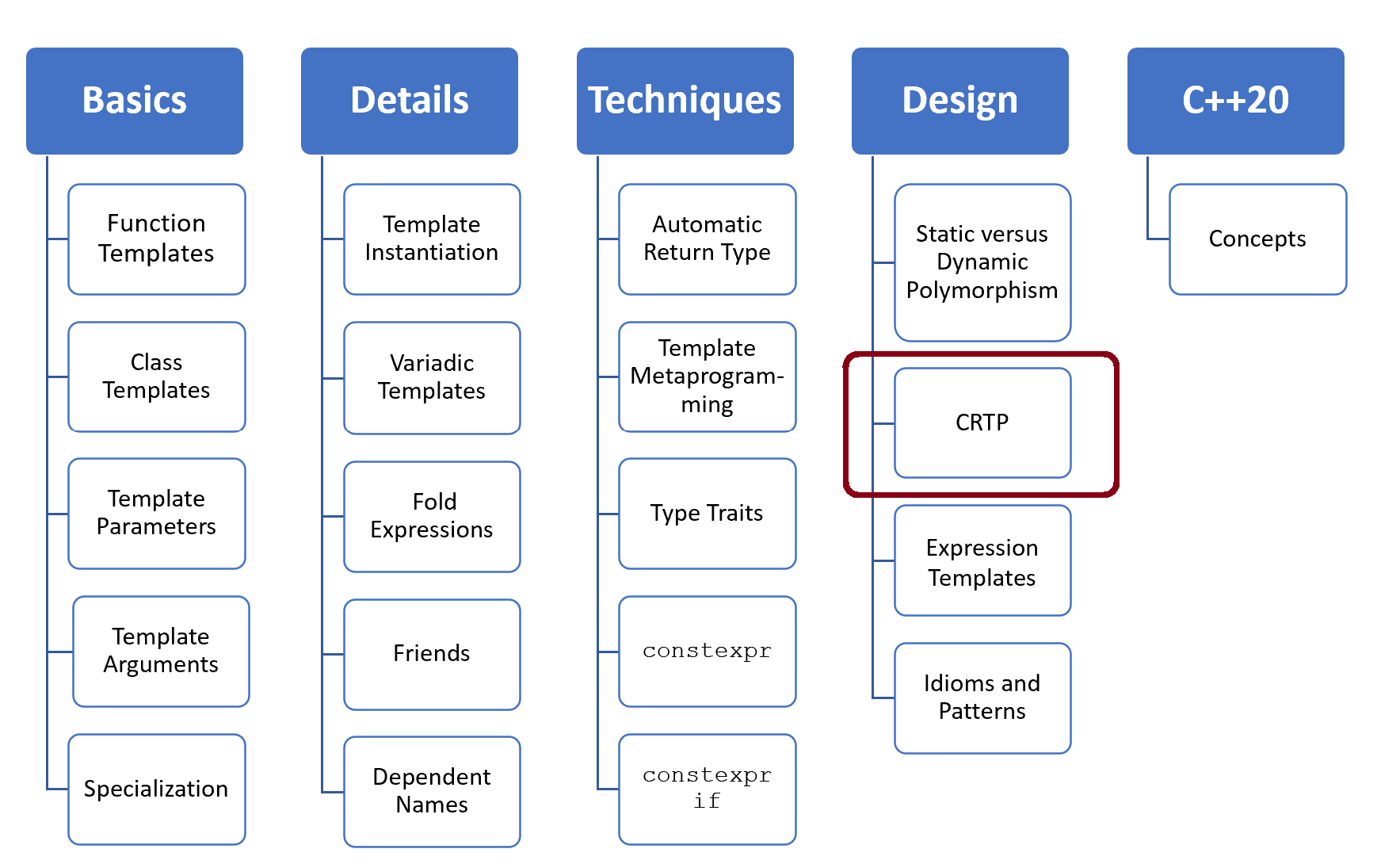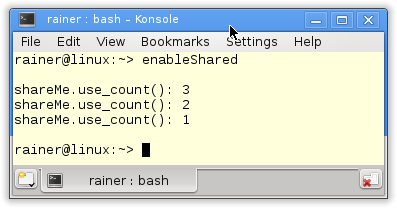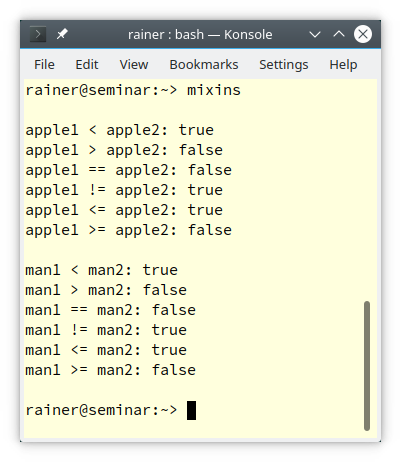# Mixins

In my previous  post “More about Dynamic and Static Polymorphism”, I used the Curiously Recurring Template Pattern (CRTP) to implement static polymorphism. Another typical use case for CRTP is mixins.Mixins are a popular idea in the design of classes to mix in new code. Therefore, it’s an often-used technique in Python to change the behavior of a class by using multiple inheritances. Contrary to C++, it is legal in Python to have more than one method definition in a class hierarchy. Python uses that method that is first in the Method Resolution Order (MRO).

You can implement mixins in C++ by using CRTP. A prominent example is the class` std::enable_shared_from_this`.

## `std::enable_shared_from_this`

Let’s see `std::enable_shared_from` this and, therefore, CRTP applied.

##Modernes C++ Mentoring

Be part of my mentoring programs:

• "Fundamentals for C++ Professionals" (open)
• "Design Patterns and Architectural Patterns with C++" (open)
• "C++20: Get the Details" (reopens December 2023)
• Do you want to stay informed about my mentoring programs: Subscribe via E-Mail.

```// enableShared.cpp

#include <iostream>
#include <memory>

class ShareMe: public std::enable_shared_from_this<ShareMe> {  // (1)
public:
std::shared_ptr<ShareMe> getShared(){
return shared_from_this();                                  // (2)
}
};

int main(){

std::cout << '\n';

std::shared_ptr<ShareMe> shareMe(new ShareMe);
std::shared_ptr<ShareMe> shareMe1= shareMe->getShared();      // (3)
{
auto shareMe2(shareMe1);
std::cout << "shareMe.use_count(): "  << shareMe.use_count() << '\n';
}
std::cout << "shareMe.use_count(): "  << shareMe.use_count() << '\n';

shareMe1.reset();

std::cout << "shareMe.use_count(): "  << shareMe.use_count() << '\n';

std::cout << '\n';

}
```

By using the class, `std::enable_shared_from_this` you can create objects that return a  `std::shared_ptr` to itself. You must derive your class public from (line 1) to get it. Now, your class `MySharedClass` has a member function` shared_from_this` (line 2) for creating `std::shared_ptr` to its objects. The call `shareMe->getShared()` (line 3) creates a new smart pointer. The member function `getShared` internally uses the function `shared_from_this` (line 2). The following screenshot shows the creation of the shared pointer.How do mixin classes work in C++? Let me introduce a typical use case.

## Extending a Class with all Relational Operators

Imagine you want to implement all six comparison operators for your data type.  (Of course, with C++20, the compiler can auto-generate them:  C++20: The Three-Way Comparison Operator). So far, you have only implemented the smaller operator (<). You know that you can derive all other five comparison operators (`<=, >, >=, ==` , and` !=`) from the smaller operator. Applying this idea and using CRTP save you many keyboard strokes.

```// mixins.cpp

#include <iostream>
#include <string>

template <typename Derived>
struct Relational {
friend bool operator > (Derived const& op1, Derived const& op2){
return op2 < op1;
}
friend bool operator == (Derived const& op1, Derived const& op2){
return !(op1 < op2) && !(op2 < op1);
}
friend bool operator != (Derived const& op1, Derived const& op2){
return (op1 < op2) || (op2 < op1);
}
friend bool operator <= (Derived const& op1, Derived const& op2){
return (op1 < op2) || (op1 == op2);
}
friend bool operator >= (Derived const& op1, Derived const& op2){
return (op1 > op2) || (op1 == op2);
}
};

class Apple: public Relational<Apple>{
public:
explicit Apple(int s): size{s}{};
friend bool operator < (Apple const& a1, Apple const& a2){    // (1)
return a1.size < a2.size;
}
private:
int size;
};

class Man: public Relational<Man>{                                 // (3)
public:
explicit Man(const std::string& n): name{n}{}
friend bool operator < (Man const& m1, Man const& m2){         // (2)
return m1.name < m2.name;
}
private:
std::string name;
};

int main(){

std::cout << std::boolalpha << '\n';

Apple apple1{5};
Apple apple2{10};
std::cout << "apple1 < apple2: " << (apple1 < apple2) << '\n';
std::cout << "apple1 > apple2: " << (apple1 > apple2) << '\n';
std::cout << "apple1 == apple2: " << (apple1 == apple2) << '\n';
std::cout << "apple1 != apple2: " << (apple1 != apple2) << '\n';
std::cout << "apple1 <= apple2: " << (apple1 <= apple2) << '\n';
std::cout << "apple1 >= apple2: " << (apple1 >= apple2) << '\n';

std::cout << '\n';

Man man1{"grimm"};
Man man2{"jaud"};
std::cout << "man1 < man2: " << (man1 < man2) << '\n';
std::cout << "man1 > man2: " << (man1 > man2) << '\n';
std::cout << "man1 == man2: " << (man1 == man2) << '\n';
std::cout << "man1 != man2: " << (man1 != man2) << '\n';
std::cout << "man1 <= man2: " << (man1 <= man2) << '\n';
std::cout << "man1 >= man2: " << (man1 >= man2) << '\n';

std::cout << '\n';

}
```

I’ve for `Apple` and `Man` implemented the smaller operator (lines 1 and 2).  For simplicity, I only use the class `Man` in my argumentation.  Man is `public` derived from the class `Relational<Man>` (line 3) using CRTP. The class `Relational` supports the five missing relational operators (`<=, <,>=, ==`, and` !=`).  The five relations operators are mapped onto the less operator of Man (line 2).Honestly, I like the name mixins for this idiom. The class `Relational` mixes the remaining relational operators into the class `Man`.

The characteristic of CRTP is that a class `Derived` derives from a class template `Base` and `Base` has `Derived` as a template argument:

```class Derived : public Base<Derived>      // (1)
{
...
};

class Derivedwrong : public Base<Derived> // (2)
{
...
};
```

How can you ensure that you are not erroneously derived the wrong class `DervivedWrong `from `Base<Derived>` such as in line 2?

## Checked CRTP

The trick is straightforward: make the constructor of `Base` private.

```// crtpCheck.cpp

#include <iostream>

template <typename Derived>
struct Base{
void interface(){
static_cast<Derived*>(this)->implementation();
}
private:
Base() = default;             // (2)
friend Derived;               // (2)
};

struct Derived1: Base<Derived1>{
void implementation(){
std::cout << "Implementation Derived1" << '\n';
}
};

struct Derived2: Base<Derived1>{   // (3)
void implementation(){
std::cout << "Implementation Derived1" << '\n';
}
};

template <typename T>
void execute(T& base){
base.interface();
}

int main(){

std::cout << '\n';

Derived1 d1;
execute(d1);

Derived2 d2;
execute(d2);

std::cout << '\n';

}
```

`Base` has a private default constructor (line 1). Only the class `Base` itself or the friend `Derived` (line 2) can invoke the default constructor. Consequentially, the call` Derived2 d2` (line 3) fails because` Derived2` it is derived from `Base<Derived1>`. Here is the error message from GCC:

## What’s next?

The Curiously Recurring Template Pattern (CRTP) is not easy to understand but very powerful. The same holds for expression templates. Expression templates allow you to get rid of superfluous temporaries.

Thanks a lot to my Patreon Supporters: Matt Braun, Roman Postanciuc, Tobias Zindl, G Prvulovic, Reinhold Dröge, Abernitzke, Frank Grimm, Sakib, Broeserl, António Pina, Sergey Agafyin, Андрей Бурмистров, Jake, GS, Lawton Shoemake, Jozo Leko, John Breland, Venkat Nandam, Jose Francisco, Douglas Tinkham, Kuchlong Kuchlong, Robert Blanch, Truels Wissneth, Kris Kafka, Mario Luoni, Friedrich Huber, lennonli, Pramod Tikare Muralidhara, Peter Ware, Daniel Hufschläger, Alessandro Pezzato, Bob Perry, Satish Vangipuram, Andi Ireland, Richard Ohnemus, Michael Dunsky, Leo Goodstadt, John Wiederhirn, Yacob Cohen-Arazi, Florian Tischler, Robin Furness, Michael Young, Holger Detering, Bernd Mühlhaus, Matthieu Bolt, Stephen Kelley, Kyle Dean, Tusar Palauri, Dmitry Farberov, Juan Dent, George Liao, Daniel Ceperley, Jon T Hess, Stephen Totten, Wolfgang Fütterer, Matthias Grün, Phillip Diekmann, Ben Atakora, Ann Shatoff, Rob North, Bhavith C Achar, and Marco Parri Empoli.

Thanks, in particular, to Jon Hess, Lakshman, Christian Wittenhorst, Sherhy Pyton, Dendi Suhubdy, Sudhakar Belagurusamy, Richard Sargeant, Rusty Fleming, John Nebel, Mipko, Alicja Kaminska, Slavko Radman, and David Poole.

 My special thanks to EmbarcaderoMy special thanks to PVS-StudioMy special thanks to Tipi.buildMy special thanks to Take Up Code## Seminars

I’m happy to give online seminars or face-to-face seminars worldwide. Please call me if you have any questions.

### Standard Seminars (English/German)

Here is a compilation of my standard seminars. These seminars are only meant to give you a first orientation.

• C++ – The Core Language
• C++ – The Standard Library
• C++ – Compact
• C++11 and C++14
• Concurrency with Modern C++
• Design Pattern and Architectural Pattern with C++
• Embedded Programming with Modern C++
• Generic Programming (Templates) with C++

#### New

• Clean Code with Modern C++
• C++20

### Contact Me

Modernes C++ Mentoring,Tags:
0 replies